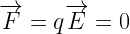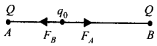Enlightened

# Question 32: NCERT Class 12th Physics: Chapter 1 – Electric Charges And Fields

• 0

Question 32: NCERT Class 12th Physics: Chapter 1 – Electric Charges And Fields

(a) Consider an arbitrary electrostatic field configuration. A small test charge is placed at a null point (i.e., where E = o) of the configuration. Show that the equilibrium of the test charge is necessarily unstable, (b) Verify this result for the simple configuration of two charges of the same magnitude and sign placed at certain distance apart.

Share

1. Solution:
(a) Let us assume that a radial electric field is present with origin as centre. The E is 0 at origin and a small test charge ‘q0’ is placed at origin. Force on test charge at origin is zero.But as the test charge is displaced a little, the electrostatic force will drift it further away so charge is in unstable equilibrium.(b) A test charge placed at mid point of equal charges is in stable equilibrium, for lateral displacement. Initially at mid point position of test charge, two repulsion forces on test charge are equal and opposite.

But on displacing q0 close to one of the charge, one of the forces become stronger and pushes the charge towards another.FA > FB so q0 moves towards B.

Check the complete chapter with solutions.

NCERT Solutions for 12th Class Physics: Chapter 1-Electric Charges And Fields

• 0